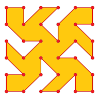# Resources tagged with: Making and proving conjectures

Filter by: Content type:
Age range:
Challenge level:

### There are 30 results

Broad Topics > Thinking Mathematically > Making and proving conjectures### Loopy

##### Age 14 to 16Challenge Level

Investigate sequences given by $a_n = \frac{1+a_{n-1}}{a_{n-2}}$ for different choices of the first two terms. Make a conjecture about the behaviour of these sequences. Can you prove your conjecture?### Multiplication Square

##### Age 14 to 16Challenge Level

Pick a square within a multiplication square and add the numbers on each diagonal. What do you notice?### Regular Hexagon Loops

##### Age 11 to 14Challenge Level

Make some loops out of regular hexagons. What rules can you discover?### Multiplication Arithmagons

##### Age 14 to 16Challenge Level

Can you find the values at the vertices when you know the values on the edges of these multiplication arithmagons?### Janine's Conjecture

##### Age 14 to 16Challenge Level

Janine noticed, while studying some cube numbers, that if you take three consecutive whole numbers and multiply them together and then add the middle number of the three, you get the middle number. . . .### Triangles Within Pentagons

##### Age 14 to 16Challenge Level

Show that all pentagonal numbers are one third of a triangular number.### Spaces for Exploration

##### Age 11 to 14

Alf Coles writes about how he tries to create 'spaces for exploration' for the students in his classrooms.### Always a Multiple?

##### Age 11 to 14Challenge Level

Think of a two digit number, reverse the digits, and add the numbers together. Something special happens...##### Age 14 to 16Challenge Level

Explore the relationship between quadratic functions and their graphs.### What's Possible?

##### Age 14 to 16Challenge Level

Many numbers can be expressed as the difference of two perfect squares. What do you notice about the numbers you CANNOT make?### Consecutive Negative Numbers

##### Age 11 to 14Challenge Level

Do you notice anything about the solutions when you add and/or subtract consecutive negative numbers?### Dice, Routes and Pathways

##### Age 5 to 14

This article for teachers discusses examples of problems in which there is no obvious method but in which children can be encouraged to think deeply about the context and extend their ability to. . . .##### Age 7 to 14Challenge Level

I added together some of my neighbours' house numbers. Can you explain the patterns I noticed?### Triangles Within Squares

##### Age 14 to 16Challenge Level

Can you find a rule which relates triangular numbers to square numbers?### Triangles Within Triangles

##### Age 14 to 16Challenge Level

Can you find a rule which connects consecutive triangular numbers?### How Old Am I?

##### Age 14 to 16Challenge Level

In 15 years' time my age will be the square of my age 15 years ago. Can you work out my age, and when I had other special birthdays?### DOTS Division

##### Age 14 to 16Challenge Level

Take any pair of two digit numbers x=ab and y=cd where, without loss of generality, ab > cd . Form two 4 digit numbers r=abcd and s=cdab and calculate: {r^2 - s^2} /{x^2 - y^2}.### To Prove or Not to Prove

##### Age 14 to 18

A serious but easily readable discussion of proof in mathematics with some amusing stories and some interesting examples.### A Little Light Thinking

##### Age 14 to 16Challenge Level

Here is a machine with four coloured lights. Can you make two lights switch on at once? Three lights? All four lights?### Maxagon

##### Age 11 to 14Challenge Level

What's the greatest number of sides a polygon on a dotty grid could have?### Exploring Simple Mappings

##### Age 11 to 14Challenge Level

Explore the relationship between simple linear functions and their graphs.### Multiple Surprises

##### Age 11 to 16Challenge Level

Sequences of multiples keep cropping up...### Problem Solving, Using and Applying and Functional Mathematics

##### Age 5 to 18Challenge Level

Problem solving is at the heart of the NRICH site. All the problems give learners opportunities to learn, develop or use mathematical concepts and skills. Read here for more information.### Polycircles

##### Age 14 to 16Challenge Level

Show that for any triangle it is always possible to construct 3 touching circles with centres at the vertices. Is it possible to construct touching circles centred at the vertices of any polygon?### Close to Triangular

##### Age 14 to 16Challenge Level

Drawing a triangle is not always as easy as you might think!### An Introduction to Magic Squares

##### Age 7 to 16### Curvy Areas

##### Age 14 to 16Challenge Level

Have a go at creating these images based on circles. What do you notice about the areas of the different sections?##### Age 14 to 16Challenge Level